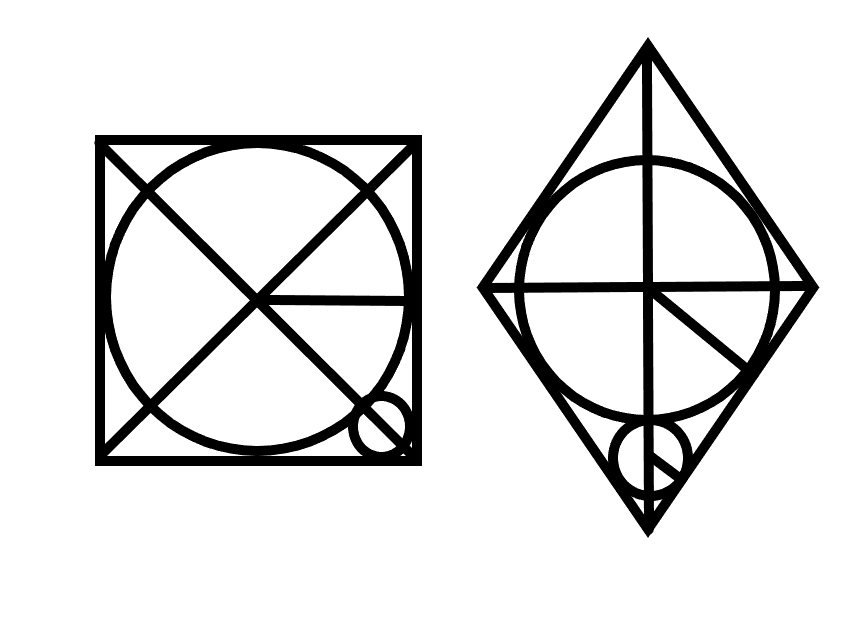# 问题 A: 你的名字

## 题目描述

Walfy同时喜欢上了n个妹子，第i个妹子的智力值为ai魅力值为bi。为了防止妹子们发现walfy是个渣男，现在walfy要给妹子排个序，这样他能按照这个排序分配和妹子聊天的时间。排序规则如下：智力高的妹子需要花费更多的时间来聊天，如果智力一样，那么魅力高的花费的时间将会多一点；如果她们的魅力也一样，姓名字典序更小的花费的时间也将多一点。

## 输入

a第一行输入一个正整数T，表示测试用例的数量。接下来是测试用例。

## 样例输入

``````3
2
abb 2 1
ac 1 100
3
abb 1 1
accb 1 1
acc 2 1
3
abb 1 1
ac 1 1
accb 1 1
``````

## 样例输出

``````1 2
2 3 1
1 2 3
``````

## 代码：

``````#include<bits/stdc++.h>
using namespace std;
struct node
{
int zs,ml;
string name;
int num2,num1;
}a;
int comp(node a,node b)
{
return a.num1<b.num1;
}
int main()
{
int t,n;
cin>>t;
while(t--)
{
cin>>n;
for(int i=1;i<=n;i++)
{
cin>>a[i].name>>a[i].zs>>a[i].ml;
a[i].num1=i;
}
for(int i=1;i<=n;i++)
for(int j=i+1;j<=n;j++)
{
if(a[i].zs<a[j].zs)swap(a[i],a[j]);
else if(a[i].zs==a[j].zs)
{
if(a[i].ml<a[j].ml)swap(a[i],a[j]);
else if(a[i].ml==a[j].ml)
if(a[i].name>a[j].name)swap(a[i],a[j]);
}
}
for(int i=1;i<=n;i++)a[i].num2=i;
sort(a+1,a+1+n,comp);
for(int i=1;i<=n;i++)cout<<a[i].num2<<" ";
cout<<endl;
}
}``````

## 样例输入

``````【输入样例1】
2
【输入样例2】
7
``````

## 样例输出

``````【输出样例1】
1
【输出样例2】
144``````

## 代码：

``````#include<bits/stdc++.h>
using namespace std;
long long a,x;
int main()
{
while(cin>>x)
{
memset(a,0,sizeof(a));
a=1,a=1;
if(x==1)
cout<<1<<endl;
else if(x==2)
cout<<1<<endl;
else if(x>2)
{
for(int i=3; i<=x; i++)
{
for(int j=1; j<=i-1; j++)
{
a[i]=a[i]+a[i-j]*j;
}
}
cout<<a[x]<<endl;
}
}
}``````

# 问题 C: Brain Power

## 题目描述

Ulire沉迷Brain Power以后，见到啥都想先O-oooooooooo AAAAE-A-A-I-A-U- JO-oooooooooooo AAE-O-A-A-U-U-A- E-eee-ee-eee AAAAE-A-E-I-E-A- JO-ooo-oo-oo-oo EEEEO-A-AAA-AAAA一下。

1）   删除字符串中除了元音字母和字母‘j’（不区分大小写）以外的所有字符。

2）   对于连续出现的字符（不区分大小写），第一个字母须为大写，其余字母须为小写；如果这个字符恰好连续出现了4次，则这4个字母都要为大写。

3）   对于连续出现的 ‘j’（不区分大小写），只保留第一个。

## 样例输入

``````1
aaaaaeeeeei
``````

## 样例输出

``AaaaaEeeeeI``

## 题解：

``````#include<bits/stdc++.h>
using namespace std;
string str1,str2;
int t;
int main()
{
cin>>t;
while(t--)
{
cin>>str1;
int n=str1.size();
int k=0;
str2.clear();
for(int i=0;i<n;i++)
{
if(str1[i]=='a'||str1[i]=='A'||str1[i]=='e'||str1[i]=='E'||str1[i]=='i'||str1[i]=='I'||str1[i]=='o'||str1[i]=='O'||str1[i]=='u'||str1[i]=='U'||str1[i]=='j'||str1[i]=='J')
//str2+=str1[i];
str2.push_back(str1[i]);
}
for(int i=0;i<str2.size();i++)
{
int sum=0;
if(str2[i]=='j'||str2[i]=='J')
{
int j=i;
while(str2[j]=='j'||str2[j]=='J')
{
sum++;
j++;
}
if(str2[i]>='a'&&str2[i]<='z')
{
str2[i]=str2[i]-32;
cout<<str2[i];
}
else cout<<str2[i];
i=i+sum-1;
}
else if(str2[i]=='a'||str2[i]=='A'||str2[i]=='e'||str2[i]=='E'||str2[i]=='i'||str2[i]=='I'||str2[i]=='o'||str2[i]=='O'||str2[i]=='u'||str2[i]=='U')
{
int f=i;
while((str2[f]==str2[i]||(str2[f]-32)==str2[i]||(str2[f]+32)==str2[i]))
{
sum++;
f++;
}
if(str2[i]>='a'&&str2[i]<='z')
{
str2[i]=str2[i]-32;
cout<<str2[i];
}
else cout<<str2[i];
if(sum>1)
{
if(sum==4)
{
if(str2[i]>='a'&&str2[i]<='z')str2[i]=str2[i]+32;
for(int f=1; f<4; f++)cout<<str2[i];
}
else
{
f=i+1;
while((str2[f]==str2[i]||(str2[f]-32)==str2[i]||(str2[f]+32)==str2[i]))
{
if(str2[f]>='A'&&str2[f]<='Z')
{
str2[f]=str2[f]+32;
cout<<str2[f];
}
else cout<<str2[f];
f++;
}
}
}
i=i+sum-1;
}
}
cout<<endl;
}
}``````

# 问题 D: NotNight打区域赛

## 输入

(1<= T <= 100000, 1 <= k <= n <= 100000)

4

10 1

10 2

10 3

10 4

jin

yin

yin

tong

## 代码：

``````#include<stdio.h>
int main()
{
int b,a,t;
scanf("%d",&t);
while(t--)
{
scanf("%d%d",&a,&b);
double x,y,z;
x=0.1*a;
y=0.2*a;
z=0.3*a;
if(x>(int)x)x=(int)x+1;
if(y>(int)y)y=(int)y+1;
if(z>(int)z)z=(int)z+1;
if(b<=x)printf("jin\n");
else if(b>x&&b<=x+y)printf("yin\n");
else if(b>x+y&&b<=x+y+z)printf("tong\n");
else printf("tie\n");
}
}``````

# 问题 E: 快乐的duxing201606

## 题目描述

duxing201606来到了教室，看到旁边坐了一个妹子。duxing201606想要搭讪，于是duxing201606灵机一动，设计出了一个游戏。现在他在纸上画了一个半径为x的圆，妹子和duxing201606轮流在圆中画长y宽z的长方形（长方形是可以任意角度画上去的，画的长方形不能重合），谁画不下长方形谁就输了。作为游戏设计者，duxing201606想要知道在双方都使用最优策略的情况下，谁会获胜。妹子先画，duxing201606后画。

## 代码：

``````#include<bits/stdc++.h>
using namespace std;
int y,z;
int x;
int main()
{
cin>>x>>y>>z;
float yuan=3.14*x*x;
int zhen=y*z;
if(y>=x*2||z>=x*2)cout<<"forever loney"<<endl;
else cout<<"clever duxing201606"<<endl;
}``````

# 问题I：送温暖

6

2 2 5 5 8 9

1

## 代码：

（深搜）

``````#include<bits/stdc++.h>
using namespace std;
const int INF=0x7f;
int n,ans,sum;
int w;
void dfs(int dep,int cnt)
{
if(dep==n)
{
ans=min(ans,abs(sum-2*cnt));
return;
}
dfs(dep+1,cnt+w[dep]);
dfs(dep+1,cnt);
}
int main()
{
scanf("%d",&n);
sum=0;
for(int i=1;i<=n;i++)
{
scanf("%d",&w[i]);
sum+=w[i];
}
ans=INF;
dfs(0,0);
printf("%d\n",ans);
}``````
``````#include<bits/stdc++.h>
using namespace std;
int w,n;
int dp;
int sum=0;
int main()
{
memset(dp,0,sizeof(dp));
scanf("%d",&n);
for(int i=1;i<=n;i++)
{
scanf("%d",&w[i]);
sum+=w[i];
}
for(int i=1;i<=n;i++)
for(int j=sum/2;j>=w[i];j--)
{
dp[j]=max(dp[j],dp[j-w[i]]+w[i]);
}
printf("%d\n",sum-dp[sum/2]*2);
return 0;
}``````

# 问题 J: 圈圈

## 题目描述

Ulire有一块菱形木板，他想在上面切下两个圆，要求在第一个圆尽可能大的前提下让第二个圆也尽可能大。Ulire显然是知道怎么切的，但他现在沉迷于Brain Power无法自拔，就把问题委托给了你。

## 样例输入

``````2 2
``````

## 样例输出

``0.1213``

## 题解：2、在后面加上*1.0

## 代码：

``````#include<bits/stdc++.h>
using namespace std;
int a,b;
double R,rx,ry,t,x,y;
int main()
{
scanf("%d%d",&a,&b);
x=a/2.0;
y=b/2.0;
t=sqrt((double)(x*x+y*y));
R=x*y/t;
rx=(x-R)/(x+R)*R;
ry=(y-R)/(y+R)*R;
printf("%.4lf\n",max(rx,ry));
}``````

# 问题 M: 签到

## 题目描述

Ulire最近在研究哈夫曼树，一种功能是将一串字符压缩为更短的01串的数据结构，现在他发现因为写错了一个条件，导致输出的所有0都变成了1，所有1都变成了0，所以他希望你能够把这个01串变回正确的形式，当然，为了精确，他每次只会给出一个字母对应的01串，所以每个01串不会超过5位。

## 样例输入

``````5
11010``````

## 样例输出

``00101``

c++语言基础题

## 代码：

``````#include<bits/stdc++.h>
using namespace std;
string s,n;
int main()
{
cin>>n;
cin>>s;
for(int i=0;i<s.size();i++)
{
if(s[i]=='1')s[i]='0';
else if(s[i]=='0')s[i]='1';
}
cout<<s<<endl;
} ``````

### python（八）——获取路径下所有子目录，或非目录子文件_柚芷的博客-程序员宝宝_python 获取子目录下的所有文件

def file_name(file_dir): '''获取路径下所有子目录、或非目录子文件''' print('a') for root, dirs, files in os.walk(file_dir): print(root) #当前目录路径 print(dirs) #当前路径下所有子目录 ...

### 大数据BI工具Tableau学习【第一期】：Tableau简介和安装_张国荣家的弟弟的博客-程序员宝宝_bi工具tableau

tableau 使命：tableau是一个可视化分析平台，也可以是一种辅助性决策的工具软件，它改变了我们使用数据解决问题的方式，使个人和组织能够充分利用自己的数据。它的能力1.tableau帮助个人和组织提供数据驱动水平2.凭借人人可用的直观可视化分析，打破了商业智能行业的原有格局3.tableau帮助人们使用数据推动变革客户现身说法...

### 统计一个字符串中每种字符出现的次数 ?_Sword_meaning的博客-程序员宝宝

var str="HELLOWORLD" ; var arr=[] ; for(var i=0 ;i&lt;str.length ;i++){ var char=str[i] ; //console.log(char) ; //通过打印char我们得到的是下面这样一个关联数组 ; ![](https://img-blog.csdnimg.cn/20191109153203371.pn...

### Leetcode--计算一个字符串中最长的有效括号字符长度_你听的到、的博客-程序员宝宝

Leetcode–计算一个字符串中最长的有效括号字符长度首先这又是一道括号匹配的问题，我这里先记录一下之前几道类似的题目：1、给定一个只包括 ‘(’，’)’，’{’，’}’，’[’，’]’ 的字符串，判断字符串是否有效。有效字符串需满足：左括号必须用相同类型的右括号闭合。左括号必须以正确的顺序闭合。注意空字符串可被认为是有效字符串。思路：首先空串题目认为是有效字符串，这里只需简单判断...

### 一个自己编写的象棋程序，可实现走棋谱（如炮二平五），工程完成度%96，跟大家分享一下，下一步要做残局，欢迎交流_NORMAN兴宇的博客-程序员宝宝

#include#include#include #includechar qipan={{'A','B','C','D','E','D','C','B','A'},{'+','+','+','+','+','+','+','+','+'},{'+','F','+','+','+','+','+','F','+'},{'G','+','G','+','G','+'

### Java面试题十五（Kafka）_夏至青柠的博客-程序员宝宝

152.kafka 可以脱离 zookeeper 单独使用吗？为什么？  kafka 不能脱离 zookeeper 单独使用，因为 kafka 使用 zookeeper 管理和协调 kafka 的节点服务器。153.kafka 有几种数据保留的策略？  kafka 有两种数据保存策略：按照过期时间保留和按照存储的消息大小保留。154.kafka 同时设置了 7 天和 10G 清除数据，到第五天的时候消息达到了 10G，这个时候 kafka 将如何处理？  这个时候 kafka 会执行数据清除工作，

### CAN中继 can总线中继器、can总线中继模块波特率和滤波设置_mmprime的博客-程序员宝宝_can中继器

can总线隔离中继器、canbridge、CanRepeater的can波特率和验收滤波设置。波特率支持2.5kbps~1Mbps之间任意设置，验收滤波设置支持20个组合的列表或屏蔽模式的ID过滤。1、配套的配置软件是绿色软件，无需安装，直接双击打开软件：2、搜索设备-读取配置3、设置can通道通讯波特率“CAN0参数”设置界面对应can总线隔离中继器的CAN1通道；“CAN1参数...